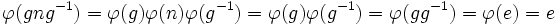# Normal subgroup equals kernel of homomorphism

This article gives the statement, and possibly proof, of a basic fact in group theory.
View a complete list of basic facts in group theory
VIEW FACTS USING THIS: directly | directly or indirectly, upto two steps | directly or indirectly, upto three steps|
This article gives a proof/explanation of the equivalence of multiple definitions for the term normal subgroup
View a complete list of pages giving proofs of equivalence of definitions

## Statement

### Verbal statement

A subgroup of a group occurs as the Kernel (?) of a group homomorphism if and only if it is normal.

### Symbolic statement

A subgroup$N$ of a group$G$ occurs as the kernel of a group homomorphism if and only if, for every$g$ in$G$,$gNg^{-1} \subseteq N$.

## Definitions used

### Kernel of a group homomorphism

A map$\varphi: G \to H$ is a homomorphism of groups if

•$\varphi(gh) = \varphi(g)\varphi(h)$ for all$g, h$ in$G$
•$\varphi(e) = e$
•$\varphi(g^{-1}) = (\varphi(g))^{-1}$

The kernel of$\varphi$ is defined as the inverse image of the identity element under$\phi$.

### Normal subgroup

For the purpose of this statement, we use the following definition of normality: a subgroup$H$ is normal in a group$G$ if$H$ contains each of its conjugate subgroups, that is,$gNg^{-1} \subseteq N$ for every$g$ in$G$.

## Related facts

Closely related to this are the isomorphism theorems.

## Proof

### Kernel of homomorphism implies normal subgroup

Let$\varphi: G \to H$ be a homomorphism of groups. We first prove that the kernel (which we call$N$) of$\phi$ is a subgroup:

• Identity element: Since$\varphi(e) = e$,$e$ is contained in$N$
• Product: Suppose$a, b$ are in$N$. Then$\varphi(a) = e$ and$\varphi(b) = e$. Using the fact that$\varphi(ab) = \varphi(a)\varphi(b)$, we conclude that$\varphi(ab) = e$. Hence$ab$ is also in$N$.
• Inverse: Suppose$a$ is in$N$. Then$\varphi(a) = e$. Using the fact that$\varphi(a^{-1}) = \varphi(a)^{-1}$, we conclude that$\varphi(a^{-1}) = e$. Hence,$a^{-1}$ is also in$N$.

Now we need to prove that$N$ is normal. In other words, we must show that if$g$ is in$G$ and$n$ is in$N$, then$gng^{-1}$ is in$N$.

Since$n$ is in$N$,$\phi(n) = e$.

Consider$\varphi(gng^{-1}) = \varphi(g)\varphi(n)\varphi(g^{-1}) = \varphi(g)\varphi(g^{-1}) = \varphi(gg^{-1}) = \varphi(e) = e$. Hence,$gng^{-1}$ must belong to$N$.

### Normal subgroup implies kernel of homomorphism

Let$N$ be a normal subgroup of a group$G$. Then,$N$ occurs as the kernel of a group homomorphism. This group homomorphism is the quotient map$\varphi: G \to G/N$, where$G/N$ is the set of cosets of$N$ in$G$.

The map is defined as follows:$\varphi(x) = xN$

Notice that the map is a group homomorphism if we equip the coset space$G/N$ with the following structure:$(aN)(bN)=abN$

This gives a well-defined group structure because, on account of$N$ being normal, the equivalence relation of being in the same coset of$N$ yields a congruence.

Explicitly:

1. The map is well-defined, because if$a' = an_1, b' = bn_2$ for$n_1,n_2 \in N$, then$a'b' = an_1bn_2 = ab(b^{-1}n_1bn_2) \in abN$ (basically, we're using that$bN = Nb$).
2. The image of the map can be thought of as a group because it satisfies associativity ($((aN)(bN))(cN) = (aN)((bN)(cN))$), has an identity element ($N$ itself), has inverses (the inverse of$aN$ is$a^{-1}N$)

Further information: quotient map